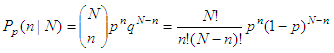# Binomial Distribution

 When a binomial distribution of events is being considered, we can use this algorithm to calculate the probability of obtaining a given number of successes in a given number of Bernoulli trials. It is necessary to provide the probability of succes on a single trial. We don't use any special statistical toolbox or function here.where
N = the number of trials
n = the exact number of successes
p = the probability of success

First, we can clear the current Matlab workspace and also the screen, to keep things clean...

clear; clc;

Then, we ask the user to enter the three required numbers... We use the 'input' function in Matlab for this purpose,

n = input('Number of trials: ');
x = input('Exact number of successes: ');
p = input('Probability of success: ');

Now, this is the main algorithm to solve the problem,

m1 = log(factorial(n));
m2 = log(factorial(x));
m3 = log(factorial(n-x));
r = exp(m1 - m2 - m3 + x*log(p) + (n-x)*log(1-p));

Finally, we display the answer (the instruction 'num2str' transforms a number into a string),

str = ['Probability of ' num2str(x) ' successes in ' ...                 num2str(n) ' trials: ' num2str(r)];
disp(str)

See the example running...

What is the probability of getting three heads in five tosses of a fair coin?

Number of trials: 5
Exact number of successes: 3
Probability of success: .5

And the Matlab response is

Probability of 3 successes in 5 trials: 0.3125

What is the probability that in five rolls of a fair die, a number 1 appears twice?

Number of trials: 5
Exact number of successes: 2
Probability of success: 1/6

And the response is

Probability of 2 successes in 5 trials: 0.16075

Reference:
Poole, L.; Borchers, M.; Some Common Basic Programs; Osborne/McGraw-Hill;
3rd. edition; Berkeley, CA; 1979.

From 'Binomial Distribution' to home

From 'Binomial Distribution' to 'Probability and Statistics'

 Top Online Calculator Normal distribution Poisson distribution F-distribution t-distribution